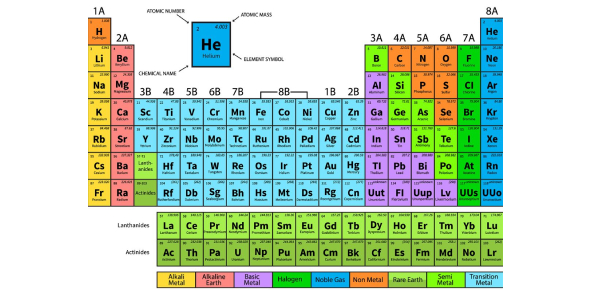Quiz: The Ultimate Periodic Table Test

10 Questions | Total Attempts: 15599SettingsThis quiz focuses on some major characteristics of the elements in the periodic table, such as groups, metals, nonmetals, metalloids, etc. This quiz consists of 10 questions to test your basic knowledge of the Periodic Table; you can also check out other science quizzes in our portal.

• 1.
How many periods are on the periodic table?
• A.

4

• B.

7

• C.

9

• 2.
What do the groups/families in the periodic table have in common?
• A.

The number of electron orbitals

• B.

The exact same characteristics

• C.

The number of valence electrons

• 3.
What do the periods on the periodic table have in common?
• A.

The number of electron orbitals

• B.

The exact same characteristics

• C.

The number of valence electrons

• 4.
The ability to pound metal into a flat sheet without breaking it is:
• A.

Luster

• B.

Malleability

• C.

Ductile

• 5.
The most reactive family of metals is the:
• A.

Transition Metals

• B.

Alkaline Earth Metals

• C.

Alkali Metals

• 6.
Which of the following is not a physical property of metals:
• A.

Luster

• B.

Corrosion

• C.

Malleability

• D.

Ductile

• 7.
Which of the following is not a characteristic of nonmetals:
• A.

Malleable

• B.

Brittle

• C.

Dull

• D.

Poor conductors of heat

• 8.
What are the elements to the left of the zigzag line called?
• A.

Nonmetals

• B.

Metalloids

• C.

Metals

• 9.
The atomic mass tells the number ____________________ in an atom.
• A.

Electrons and neutrons

• B.

Electrons and protons

• C.

Protons and neutrons

• 10.
Elements on the periodic table are ordered according to their:
• A.

Number of neutrons

• B.

Atomic mass

• C.

Atomic number

Related TopicsBack to top